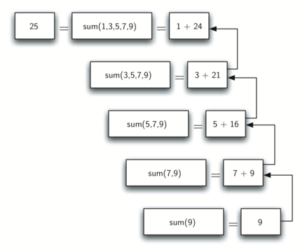TORONTO KIDS COMPUTER CLUB | Friday 17:00 Python Practice 21.02.19.
19085

# Friday 17:00 Python Practice 21.02.19.

## 23 Feb Friday 17:00 Python Practice 21.02.19.

Question:

Use the recursion we have learn in the class, please try to create a function to recursively calculate the total of 1 + 2 + …. (n-2) + (n-1) + n

`def total(n):    # please write you program belowtotal(int(input('Please enter a number:')))`

Class Notes:

All recursive algorithms must obey three important laws:

1. A recursive algorithm must have a base case.

2. A recursive algorithm must change its state and move toward the base case.

3. A recursive algorithm must call itself, recursively.

Sample program:

`def listsum(numList):    if len(numList) == 1:        return numList    else:        return numList + listsum(numList[1:])print(listsum([1,3,5,7,9]))`